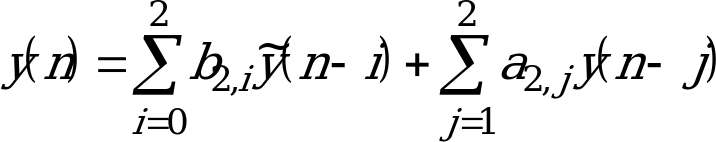## 4.1.1 High-pass filter

06.203GPPHalf rate speech transcodingTS

The 13 bit linear Pulse Code Modulated (PCM) input speech, x(n), is filtered by a fourth order pole-zero high pass filter. This filter suppresses the frequency components of the input speech which are below 120 Hz. The filter is implemented as a cascade of two second-order Infinite Impulse Response (IIR) filters. Incorporated into the filter coefficients is a gain of 0,5. The difference equation for the first filter is:

(3)

where:

b10 = 0,335052

b11 = ‑0,669983 a11 = 0,926117

b12 = 0,335052 a12 = ‑0,429413

The difference equation for the second filter is:(4)

where:

b20 = 0,335052

b21 = ‑0,669434 a21= 0,965332

b22 = 0,335052 a22 = ‑0,469513